# Maharashtra Board 11th Maths Solutions Chapter 4 Determinants and Matrices Miscellaneous Exercise 4(A)

Balbharti Maharashtra State Board Class 11 Maths Solutions Pdf Chapter 4 Determinants and Matrices Miscellaneous Exercise 4(A) Questions and Answers.

## Maharashtra State Board 11th Maths Solutions Chapter 4 Determinants and Matrices Miscellaneous Exercise 4(A)

I. Select the correct option from the given alternatives.

Question 1.
The determinant D = $$\left|\begin{array}{ccc} a & b & a+b \\ b & c & b+c \\ a+b & b+c & 0 \end{array}\right|$$ = 0, if
(a) a, b, c are in A.P.
(b) a, b, c are in G.P.
(c) a, b, c are in H.P.
(d) α is a root of ax2 + 2bx + c = 0
(b) a, b, c are in G.P.
Hint:
Applying R3 → R3 – (R1 + R2), we get
$$\left|\begin{array}{llc} a & b & a+b \\ b & c & b+c \\ 0 & 0 & -(a+2 b+c) \end{array}\right|=0$$
∴ a[-c(a + 2b + c) – 0] – b[-b(a + 2b + c) – 0] + (a + b) (0 – 0) = 0
∴ (-ac + b2) (a + 2b + c) = 0
∴ -ac + b2 = 0 or a + 2b + c = 0
∴ b2 = ac
∴ a, b, c are in G.P.Question 2.
If $$\left|\begin{array}{lll} x^{k} & x^{k+2} & x^{k+3} \\ y^{k} & y^{k+2} & y^{k+3} \\ z^{k} & z^{k+2} & z^{k+3} \end{array}\right|$$ = (x – y) (y – z) (z – x) $$\left(\frac{1}{x}+\frac{1}{y}+\frac{1}{z}\right)$$ then
(a) k = -3
(b) k = -1
(c) k = 1
(d) k = 3
(b) k = -1
Hint: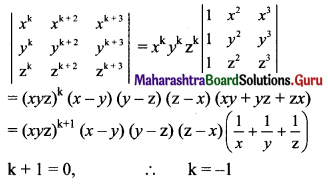Question 3.
Let D = $$\left|\begin{array}{ccc} \sin \theta \cdot \cos \phi & \sin \theta \cdot \sin \phi & \cos \theta \\ \cos \theta \cdot \cos \phi & \cos \theta \cdot \sin \phi & -\sin \theta \\ -\sin \theta \cdot \sin \phi & \sin \theta \cdot \cos \phi & 0 \end{array}\right|$$ then
(a) D is independent of θ
(b) D is independent of φ
(c) D is a constant
(d) $$\frac{d D}{d}$$ at θ = $$\frac{\pi}{2}$$ is equal to 0
(b) D is independent of φ

Question 4.
The value of a for which the system of equations a3x + (a + 1)y + (a + 2)3 z = 0, ax + (a + 1)y + (a + 2)z = 0 and x + y + z = 0 has a non zero solution is
(a) 0
(b) -1
(c) 1
(d) 2
(b) -1
Hint:
The given system of equations will have a non-zero solution, if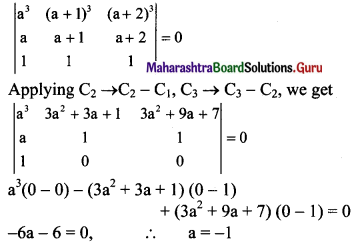Question 5.
$$\left|\begin{array}{lll} b+c & c+a & a+b \\ q+r & r+p & p+q \\ y+z & z+x & x+y \end{array}\right|=$$
(a) 2 $$\left|\begin{array}{lll} c & b & a \\ r & q & p \\ z & y & x \end{array}\right|$$
(b) 2 $$\left|\begin{array}{lll} b & a & c \\ q & p & r \\ y & x & z \end{array}\right|$$
(c) 2 $$\left|\begin{array}{lll} a & b & c \\ p & q & r \\ x & y & z \end{array}\right|$$
(d) 2 $$\left|\begin{array}{lll} a & c & b \\ p & r & q \\ x & z & y \end{array}\right|$$
(c) 2 $$\left|\begin{array}{lll} a & b & c \\ p & q & r \\ x & y & z \end{array}\right|$$
Hint: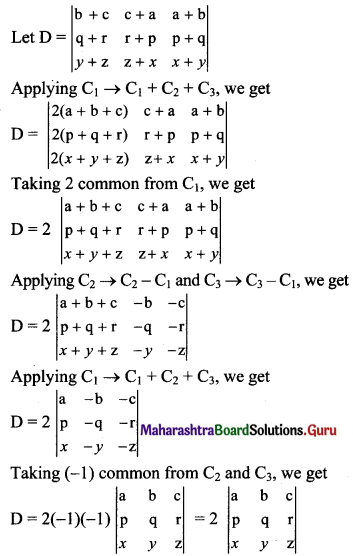Question 6.
The system 3x – y + 4z = 3, x + 2y – 3z = -2 and 6x + 5y + λz = -3 has atleast one solution when
(a) λ = -5
(b) λ = 5
(c) λ = 3
(d) λ = -13
(a) λ = -5
Hint:
The given system of equations will have more than one solution if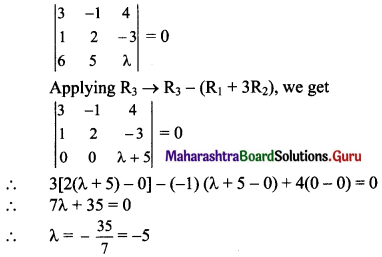Question 7.
If x = -9 is a root of $$\left|\begin{array}{lll} x & 3 & 7 \\ 2 & x & 2 \\ 7 & 6 & x \end{array}\right|=0$$, has other two roots are
(a) 2, -7
(b) -2, 7
(c) 2, 7
(d) -2, -7
(c) 2, 7
Hint: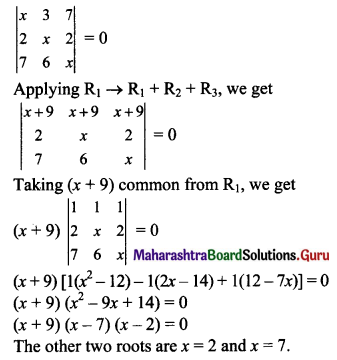Question 8.
If $$\left|\begin{array}{ccc} 6 i & -3 i & 1 \\ 4 & 3 i & -1 \\ 20 & 3 & i \end{array}\right|$$ = x + iy, then
(a) x = 3, y = 1
(b) x = 1, y = 3
(c) x = 0, y = 3
(d) x = 0, y = 0
(d) x = 0, y = 0

Question 9.
If A(0, 0), B(1, 3) and C(k, 0) are vertices of triangle ABC whose area is 3 sq.units, then the value of k is
(a) 2
(b) -3
(c) 3 or -3
(d) -2 or 2
(d) -2 or 2Question 10.
Which of the following is correct?
(a) Determinant is a square matrix
(b) Determinant is number associated to matrix
(c) Determinant is a number associated with a square matrix
(d) None of these
(c) Determinant is a number associated with a square matrix

Question 1.
Evaluate:
(i) $$\left|\begin{array}{ccc} 2 & -5 & 7 \\ 5 & 2 & 1 \\ 9 & 0 & 2 \end{array}\right|$$
(ii) $$\left|\begin{array}{ccc} 1 & -3 & 12 \\ 0 & 2 & -4 \\ 9 & 7 & 2 \end{array}\right|$$
Solution:
(i) $$\left|\begin{array}{ccc} 2 & -5 & 7 \\ 5 & 2 & 1 \\ 9 & 0 & 2 \end{array}\right|$$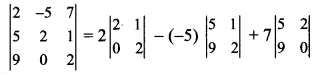= 2(4 – 0) + 5(10 – 9) + 7(0 – 18)
= 2(4) + 5(1) + 7(-18)
= 8 + 5 – 126
= -113

(ii) $$\left|\begin{array}{ccc} 1 & -3 & 12 \\ 0 & 2 & -4 \\ 9 & 7 & 2 \end{array}\right|$$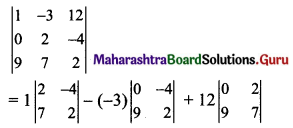= 1(4 + 28) + 3(0 + 36) + 12(0 – 18)
= 1(32) + 3(36) + 12(-18)
= 32 + 108 – 216
= -76Question 2.
Evaluate determinant along second column $$\left|\begin{array}{ccc} 1 & -1 & 2 \\ 3 & 2 & -2 \\ 0 & 1 & -2 \end{array}\right|$$
Solution: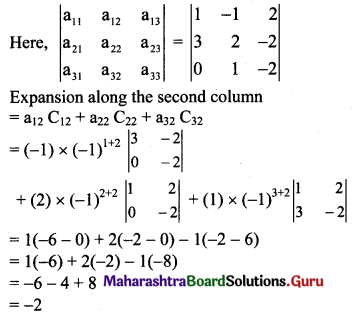Question 3.
Evaluate:
(i) $$\left|\begin{array}{ccc} 2 & 3 & 5 \\ 400 & 600 & 1000 \\ 48 & 47 & 18 \end{array}\right|$$
(ii) $$\left|\begin{array}{ccc} 101 & 102 & 103 \\ 106 & 107 & 108 \\ 1 & 2 & 3 \end{array}\right|$$
by using properties.
Solution: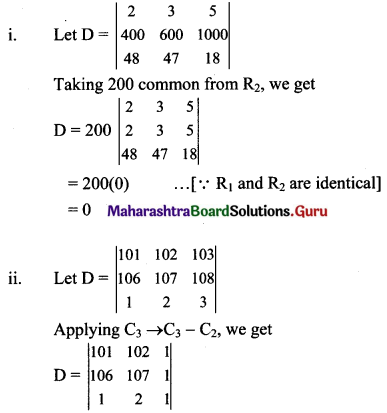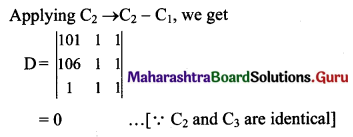Question 4.
Find the minors and cofactors of elements of the determinants.
(i) $$\left|\begin{array}{ccc} -1 & 0 & 4 \\ -2 & 1 & 3 \\ 0 & -4 & 2 \end{array}\right|$$
(ii) $$\left|\begin{array}{ccc} 1 & -1 & 2 \\ 3 & 0 & -2 \\ 1 & 0 & 3 \end{array}\right|$$
Solution: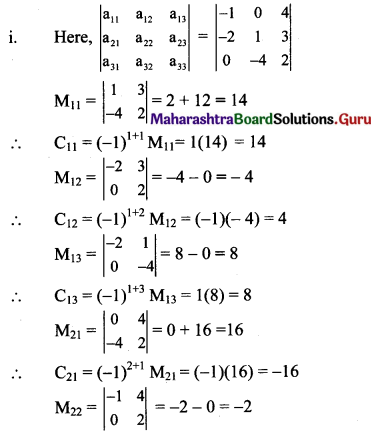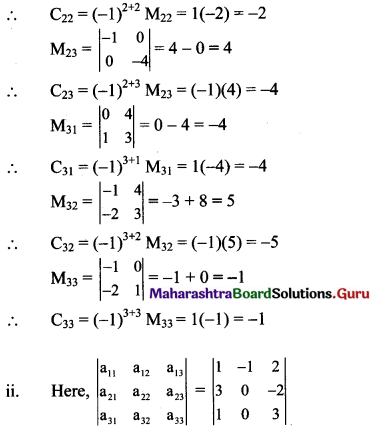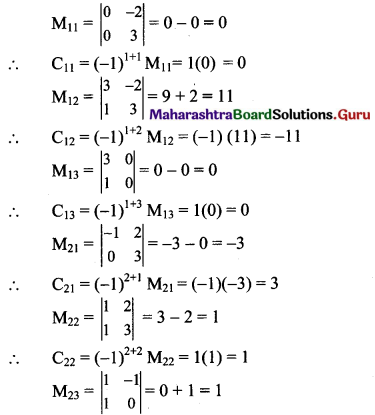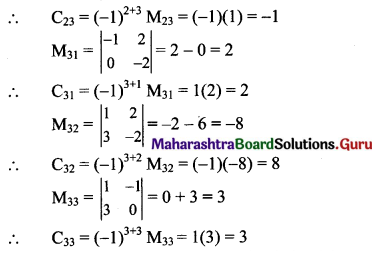Question 5.
Find the values of x, if
(i) $$\left|\begin{array}{ccc} 1 & 4 & 20 \\ 1 & -2 & -5 \\ 1 & 2 x & 5 x^{2} \end{array}\right|=0$$
(ii) $$\left|\begin{array}{ccc} 1 & 2 x & 4 x \\ 1 & 4 & 16 \\ 1 & 1 & 1 \end{array}\right|=0$$
Solution:
(i) $$\left|\begin{array}{ccc} 1 & 4 & 20 \\ 1 & -2 & -5 \\ 1 & 2 x & 5 x^{2} \end{array}\right|=0$$
⇒ 1(-10x2 + 10x) – 4(5x2 + 5) + 20(2x + 2) = 0
⇒ -10x2 + 10x – 20x2 – 20 + 40x + 40 = 0
⇒ -30x2 + 50x + 20 = 0
⇒ 3x2 – 5x – 2 = 0 …..[Dividing throughout by (-10)]
⇒ 3x2 – 6x + x – 2 = 0
⇒ 3x(x – 2) + 1(x – 2) = 0
⇒ (x – 2) (3x + 1) = 0
⇒ x – 2 = 0 or 3x + 1 = 0
⇒ x = 2 or x = $$-\frac{1}{3}$$

(ii) $$\left|\begin{array}{ccc} 1 & 2 x & 4 x \\ 1 & 4 & 16 \\ 1 & 1 & 1 \end{array}\right|=0$$
⇒ 1(4 – 16) – 2x(1 – 16) + 4x(1 – 4) = 0
⇒ 1(-12) – 2x(-15) + 4x(-3) = 0
⇒ -12 + 30x – 12x = 0
⇒ 18x = 12
⇒ x = $$\frac{12}{18}=\frac{2}{3}$$Question 6.
By using properties of determinant, prove that $$\left|\begin{array}{ccc} x+y & y+z & z+x \\ z & x & y \\ 1 & 1 & 1 \end{array}\right|=0$$
Solution: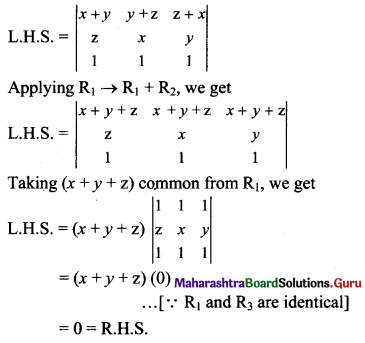Question 7.
Without expanding the determinants, show that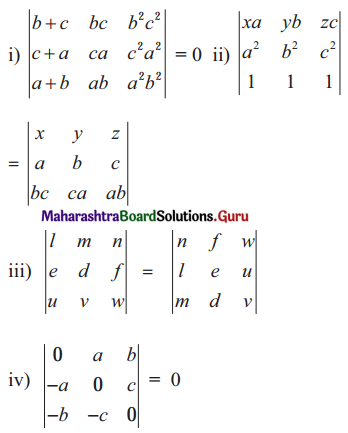Solution: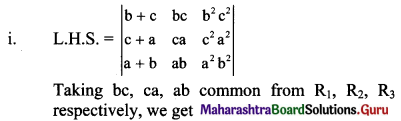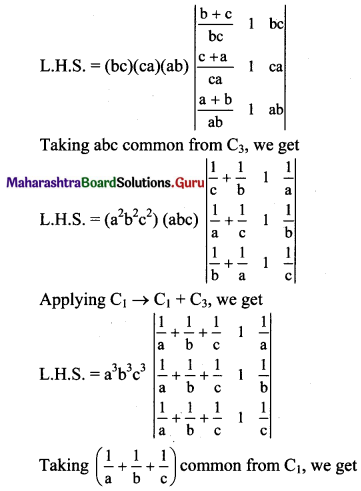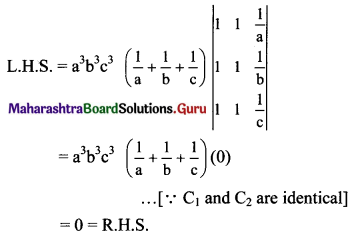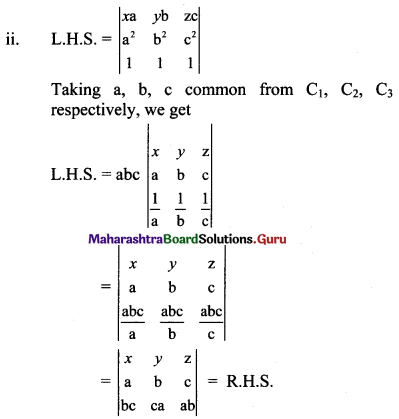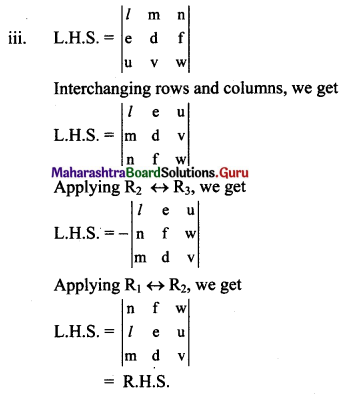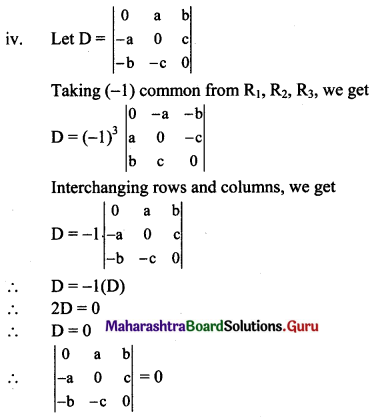Question 8.
If $$\left|\begin{array}{lll} a & 1 & 1 \\ 1 & b & 1 \\ 1 & 1 & c \end{array}\right|=0$$ then show that $$\frac{1}{1-a}+\frac{1}{1-b}+\frac{1}{1-c}=1$$
Solution: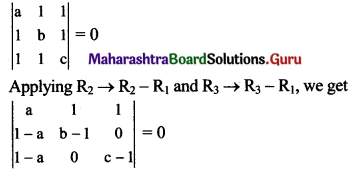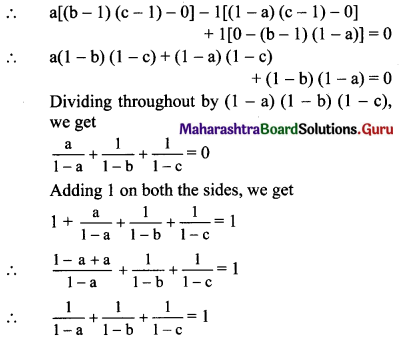Question 9.
Solve the following linear equations by Cramer’s Rule.
(i) 2x – y + z = 1, x + 2y + 3z = 8, 3x + y – 4z = 1
(ii) $$\frac{1}{x}+\frac{1}{y}=\frac{3}{2}, \quad \frac{1}{y}+\frac{1}{z}=\frac{5}{6}, \quad \frac{1}{z}+\frac{1}{x}=\frac{4}{3}$$
(iii) 2x + 3y + 3z = 5, x – 2y + z = -4, 3x – y – 2z = 3
(iv) x + y + 2z = 7, 3x + 4y – 5z = 5, 2x – y + 3z = 12
Solution:
(i) Given equations are
2x – y + z = 1
x + 2y + 3z = 8
3x + y – 4z = 1
D = $$\left|\begin{array}{ccc} 2 & -1 & 1 \\ 1 & 2 & 3 \\ 3 & 1 & -4 \end{array}\right|$$
= 2(-8 – 3) – (-1)(-4 – 9) + 1(1 – 6)
= 2(-11) + 1(-13) + 1(-5)
= -22 – 13 – 5
= -40 ≠ 0

Dx = $$\left|\begin{array}{ccc} 1 & -1 & 1 \\ 8 & 2 & 3 \\ 1 & 1 & -4 \end{array}\right|$$
= 1(-8 – 3) – (-1)(-32 – 3) + 1(8 – 2)
= 1(-11) + 1(-35) + 1(6)
= -11 – 35 + 6
= -40

Dy = $$\left|\begin{array}{ccc} 2 & 1 & 1 \\ 1 & 8 & 3 \\ 3 & 1 & -4 \end{array}\right|$$
= 2(-32 – 3) -1(-4 – 9) + 1(1 – 24)
= 2(-35) – 1(-13) + 1(-23)
= -70 + 13 – 23
= -80

Dz = $$\left|\begin{array}{ccc} 2 & -1 & 1 \\ 1 & 2 & 8 \\ 3 & 1 & 1 \end{array}\right|$$
= 2(2 – 8) – (-1)(1 – 24) + 1(1 – 6)
= 2(-6) + 1(-23) + 1(-5)
= -12 – 23 – 5
= -40
By Cramer’s Rule,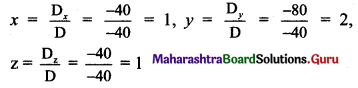∴ x = 1, y = 2 and z = 1 are the solutions of the given equations.(ii) Let $$\frac{1}{x}$$ = p, $$\frac{1}{y}$$ = q, $$\frac{1}{z}$$ = r
∴ The given equations become
p + q = $$\frac{3}{2}$$
i.e., 2p + 2q = 3
i.e., 2p + 2q + 0 = 3
q + r = $$\frac{5}{6}$$
i.e., 6q + 6r = 5,
i.e., 0p + 6q + 6r = 5
r + p = $$\frac{4}{3}$$
i.e., 3r + 3p = 4,
i.e., 3p + 0q + 3r = 4
D = $$\left|\begin{array}{lll} 2 & 2 & 0 \\ 0 & 6 & 6 \\ 3 & 0 & 3 \end{array}\right|$$
= 2(18 – 0) -2(0 – 18) + 0
= 2(18) – 2(-18)
= 36 + 36
= 72 ≠ 0

Dp = $$\left|\begin{array}{lll} 3 & 2 & 0 \\ 5 & 6 & 6 \\ 4 & 0 & 3 \end{array}\right|$$
= 3(18 – 0) – 2(15 – 24) + 0
= 3(18) – 2(-9)
= 54 + 18
= 72

Dq = $$\left|\begin{array}{lll} 2 & 3 & 0 \\ 0 & 5 & 6 \\ 3 & 4 & 3 \end{array}\right|$$
= 2(15 – 24) – 3(0 – 18) + 0
= 2(-9) – 3(-18)
= -18 + 54
= 36

Dr = $$\left|\begin{array}{lll} 2 & 2 & 3 \\ 0 & 6 & 5 \\ 3 & 0 & 4 \end{array}\right|$$
= 2(24 – 0) – 2(0 – 15) + 3(0 – 18)
= 2(24) – 2(-15) + 3(-18)
= 48 + 30 – 54
= 24
By Cramer’s Rule,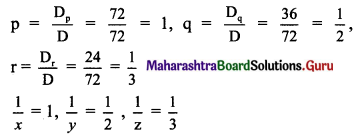∴ x = 1, y = 2 and z = 3 are the solutions of the given equations.

(iii) Given equations are
2x + 3y + 3z = 5
x – 2y + z = -4
3x – y – 2z = 3
D = $$\left|\begin{array}{ccc} 2 & 3 & 3 \\ 1 & -2 & 1 \\ 3 & -1 & -2 \end{array}\right|$$
= 2(4 + 1) – 3(-2 – 3) + 3(-1 + 6)
= 2(5) – 3(-5) + 3(5)
= 10 + 15 + 15
= 40 ≠ 0

Dx = $$\left|\begin{array}{ccc} 5 & 3 & 3 \\ -4 & -2 & 1 \\ 3 & -1 & -2 \end{array}\right|$$
= 5(4 + 1) – 3(8 – 3) + 3(4 + 6)
= 5(5) – 3(5) + 3(10)
= 25 – 15 + 30
= 40

Dy = $$\left|\begin{array}{ccc} 2 & 5 & 3 \\ 1 & -4 & 1 \\ 3 & 3 & -2 \end{array}\right|$$
= 2(8 – 3) – 5(-2 – 3) + 3(3 + 12)
= 2(5) – 5(-5) + 3(15)
= 10 + 25 + 45
= 80

Dz = $$\left|\begin{array}{ccc} 2 & 3 & 5 \\ 1 & -2 & -4 \\ 3 & -1 & 3 \end{array}\right|$$
= 2(-6 – 4) – 3(3 + 12) + 5(-1 + 6)
= 2(-10) – 3(15) + 5(5)
= -20 -45 + 25
= -40
By Cramer’s Rule,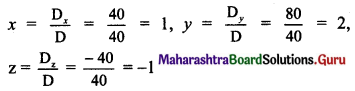∴ x = 1, y = 2 and z = -1 are the solutions of the given equations.

(iv) Given equations are
x – y + 2z = 7
3x + 4y – 5z = 5
2x – y + 3z = 12
D = $$\left|\begin{array}{ccc} 1 & -1 & 2 \\ 3 & 4 & -5 \\ 2 & -1 & 3 \end{array}\right|$$
= 1(12 – 5) – (-1)(9 + 10) + 2(-3 – 8)
= 1(7) + 1(19) + 2(-11)
= 7 + 19 – 22
= 4 ≠ 0

Dx = $$\left|\begin{array}{ccc} 7 & -1 & 2 \\ 5 & 4 & -5 \\ 12 & -1 & 3 \end{array}\right|$$
= 7(12 – 5) – (-1)(15 + 60) + 2(-5 – 48)
= 7(7) + 1(75) + 2(-53)
= 49 + 75 – 106
= 18

Dy = $$\left|\begin{array}{ccc} 1 & 7 & 2 \\ 3 & 5 & -5 \\ 2 & 12 & 3 \end{array}\right|$$
= 1(15 + 60) – 7(9 + 10) + 2(36 – 10)
= 1(75) – 7(19) + 2(26)
= 75 – 133 + 52
= -6

Dz = $$\left|\begin{array}{ccc} 1 & -1 & 7 \\ 3 & 4 & 5 \\ 2 & -1 & 12 \end{array}\right|$$
= 1(48 + 5) – (-1)(36 – 10) + 7(-3 – 8)
= 1(53) + 1(26) + 7(-11)
= 53 + 26 – 77
= 2
By Cramer’s Rule,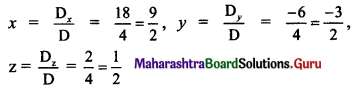∴ x = $$\frac{9}{2}$$, y = $$\frac{-3}{2}$$ and z = $$\frac{1}{2}$$ are the solutions of the given equations.Question 10.
Find the value of k, if the following equations are consistent.
(i) (k + 1)x + (k – 1)y + (k – 1) = 0
(k – 1)x + (k + 1)y + (k – 1) = 0
(k – 1)x + (k – 1)y + (k + 1) = 0
(ii) 3x + y – 2 = 0, kx + 2y – 3 = 0 and 2x – y = 3
(iii) (k – 2)x + (k – 1)y = 17, (k – 1)x +(k – 2)y = 18 and x + y = 5
Solution:
(i) Given equations are
(k + 1)x + (k – 1)y + (k – 1) = 0
(k – 1)x + (k + 1)y + (k – 1) = 0
(k – 1)x + (k – 1)y + (k + 1) = 0
Since these equations are consistent,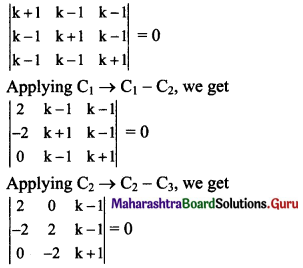⇒ 2(2k + 2 + 2k – 2) – 0 + (k – 1) (4 – 0) = 0
⇒ 2(4k) + (k – 1)4 = 0
⇒ 8k + 4k – 4 = 0
⇒ 12k – 4 = 0
⇒ k = $$\frac{4}{12}=\frac{1}{3}$$

(ii) Given equations are
3x + y – 2 = 0
kx + 2y – 3 = 0
2x – y = 3, i.e., 2x – y – 3 = 0.
Since these equations are consistent,
$$\left|\begin{array}{rrr} 3 & 1 & -2 \\ k & 2 & -3 \\ 2 & -1 & -3 \end{array}\right|=0$$
⇒ 3(-6 – 3) – 1(-3k + 6) – 2(-k – 4) = 0
⇒ 3(-9) – 1(-3k + 6) – 2(-k – 4) = 0
⇒ -27 + 3k – 6 + 2k + 8 = 0
⇒ 5k – 25 = 0
⇒ k = 5

(iii) Given equations are
(k – 2)x + (k – 1)y = 17
⇒ (k – 2)x + (k – 1)y – 17 = 0
(k – 1)x + (k – 2)y = 18
⇒ (k – 1)x + (k – 2)y – 18 = 0
x + y = 5
⇒ x + y – 5 = 0
Since these equations are consistent,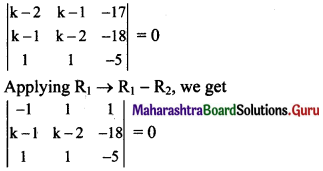⇒ -1(-5k + 10 + 18) – 1(-5k + 5 + 18) + 1(k – 1 – k + 2) = 0
⇒ -1(-5k + 28) – 1(-5k + 23) + 1(1) = 0
⇒ 5k – 28 + 5k – 23 + 1 = 0
⇒ 10k – 50 = 0
⇒ k = 5

Question 11.
Find the area of triangle whose vertices are
(i) A(-1, 2), B(2, 4), C(0, 0)
(ii) P(3, 6), Q(-1, 3), R(2, -1)
(iii) L(1, 1), M(-2, 2), N(5, 4)
Solution:
(i) Here, A(x1, y1) = A(-1, 2)
B(x2, y2) = B(2, 4)
C(x3, y3) = C(0, 0)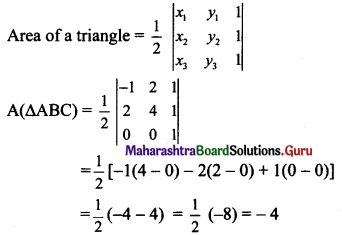Since area cannot be negative,
A(ΔABC) = 4 sq.units

(ii) Here, P(x1, y1) = P(3, 6)
Q(x2, y2) = Q(-1, 3)
R(x3, y3) = R(2, -1)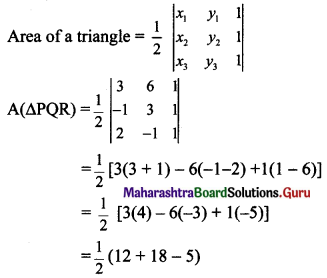A(ΔPQR) = $$\frac{25}{2}$$ sq.units

(iii) Here, L(x1, y1) = L(1, 1)
M(x2, y2) = M(-2, 2)
N(x3, y3) = N(5, 4)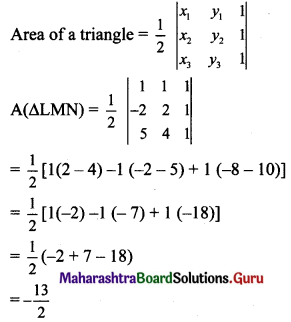Since area cannot be negative,
A(ΔLMN) = $$\frac{13}{2}$$ sq.unitsQuestion 12.
Find the value of k,
(i) if the area of a triangle is 4 square units and vertices are P(k, 0), Q(4, 0), R(0, 2).
(ii) if area of triangle is $$\frac{33}{2}$$ square units and vertices are L(3, -5), M(-2, k), N(1, 4).
Solution:
(i) Here, P(x1, y1) = P(k, 0)
Q(x2, y2) = Q(4, 0)
R(x3, y3) = R(0, 2)
A(ΔPQR) = 4 sq.units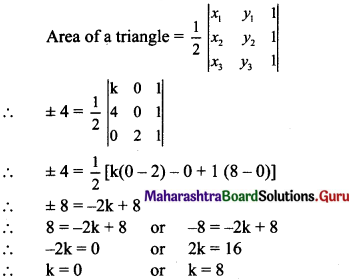(ii) Here, L(x1, y1) = L(3, -5), M(x2, y2) = M(-2, k), N(x3, y3) = N(1, 4)
A(ΔLMN) = $$\frac{33}{2}$$ sq. units
Area of a triangle = $$\frac{1}{2}\left|\begin{array}{lll} x_{1} & y_{1} & 1 \\ x_{2} & y_{2} & 1 \\ x_{3} & y_{3} & 1 \end{array}\right|$$
$$\pm \frac{33}{2}=\frac{1}{2}\left|\begin{array}{ccc} 3 & -5 & 1 \\ -2 & k & 1 \\ 1 & 4 & 1 \end{array}\right|$$
⇒ $$\pm \frac{33}{2}=\frac{1}{2}$$ [3(k – 4) – (-5) (-2 – 1) + 1 (-8 – k)]
⇒ ±33 = 3k – 12 – 15 – 8 – k
⇒ ±33 = 2k – 35
⇒ 2k – 35 = 33 or 2k – 35 = -33
⇒ 2k = 68 or 2k = 2
⇒ k = 34 or k = 1

Question 13.
Find the area of quadrilateral whose vertices are A(0, -4), B(4, 0), C(-4,0), D (0, 4).
Solution:
A(0, -4), B(4, 0), C(-4, 0), D(0, 4)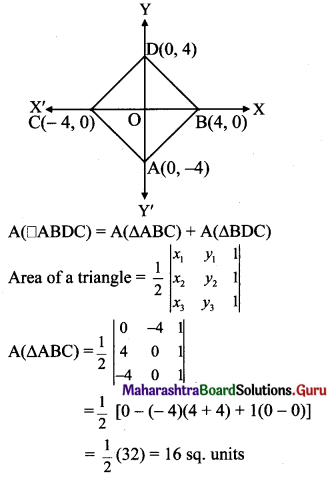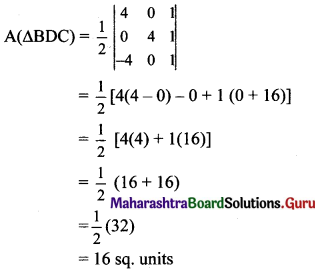∴ A(ABDC) = A(ΔABC) + A(ΔBDC)
= 16 + 16
= 32 sq.units

Question 14.
An amount of ₹ 5000 is put into three investments at the rate of interest of 6%, 7%, and 8% per annum respectively. The total annual income is ₹ 350. If the combined income from the first two investments is ₹ 70 more than the income from the third, find the amount of each investment.
Solution:
Let the amount of each investment be ₹ x, ₹ y and ₹ z.
According to the given conditions,
x + y + z = 5000,
6% x + 7% y + 8% z = 350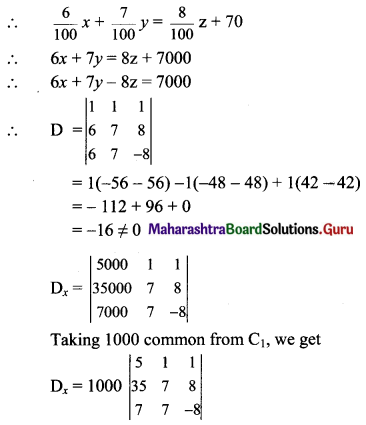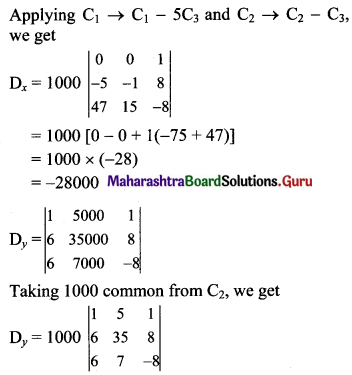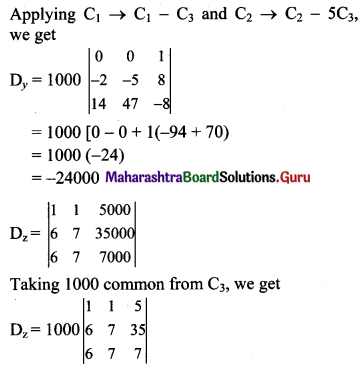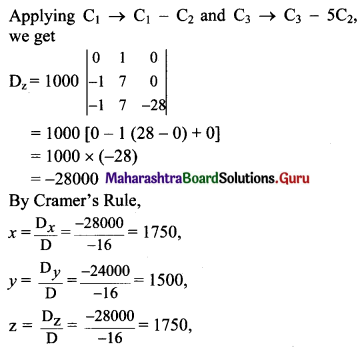∴ The amounts of investments are ₹ 1750, ₹ 1500, and ₹ 1750.Question 15.
Show that the lines x – y = 6, 4x – 3y = 20 and 6x + 5y + 8 = 0 are concurrent. Also, find the point of concurrence.
Solution:
Given equations of the lines are
x – y = 6, i.e., x – y – 6 = 0 ……(i)
4x – 3y = 20, i.e., 4x – 3y – 20 = 0 …..(ii)
6x + 5y + 8 = 0 ……(iii)
The given lines will be concurrent, if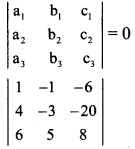= 1(-24 + 100) – (-1) (32 + 120) – 6(20 + 18)
= 1(76) + 1(152) – 6(38)
= 76 + 152 – 228
= 0
∴ The given lines are concurrent.
To find the point of concurrence, solve any two equations.
Multiplying (i) by 5, we get
5x – 5y – 30 = 0 …….(iv)
Adding (iii) and (iv), we get
11x – 22 = 0
∴ x = 2
Substituting x = 2 in (i), we get
2 – y – 6 = 0
∴ y = -4
∴ The point of concurrence is (2, -4).

Question 16.
Show that the following points are collinear using determinants:
(i) L(2, 5), M(5, 7), N(8, 9)
(ii) P(5,1), Q(1, -1), R(11, 4)
Solution:
(i) Here, L(x1, y1) = L(2, 5)
M(x2, y2) = M(5, 7)
N(X3 y3) = N(8, 9)
If A(ΔLMN) = 0, then the points L, M, N are collinear.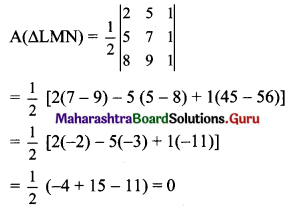∴ The points L, M, N are collinear.(ii) Here, P(x1, y1) = P(5, 1)
Q(x2, y2) = Q(1, -1)
R(x3, y3) = R(11, 4)
If A(ΔPQR) = 0, then the points P, Q, R are collinear.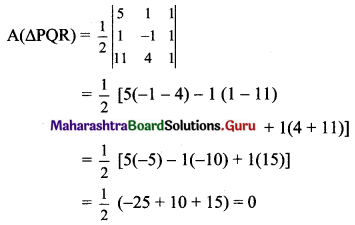∴ The points P, Q, R are collinear.